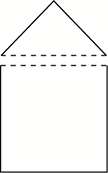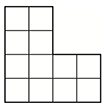Decomposition of Geometric Figures

My Definition

Key Characteristics

The following are true of decomposition of geometric figures:

• To decompose is to separate into two or more parts.

• To decompose figures can mean the separation of a shape into two or more nonoverlapping shapes.

Note: In Algebra II and subsequent coursework, the verb "decompose" refers to recognition of functions that were combined to make a new one.

Example

The pentagon below has been decomposed to create a square and a triangle.With this tool, you can explore how to decompose shapes and recompose them to make other shapes.

Non-example

The composite figure below is composed of two rectangles.The following figures are overlapping, and as such are not a decomposition of figures.Click on the link below to further study examples of composition of geometric figures.

Composition of Geometric Figures Activity

TEKS: 2(8)(E), 3(6)(D), 3(6)(E), 5(4)(A), 7(9)(C)